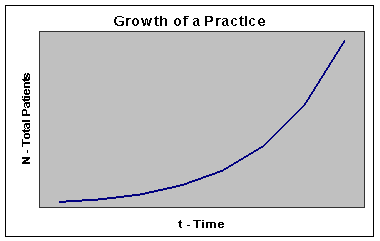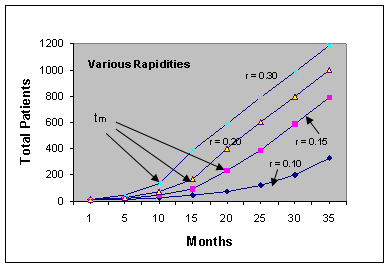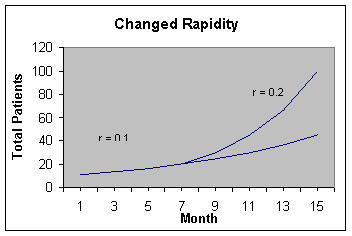RAPIDITY ANALYSIS

How Rapidly is Your Practice Growing?

In establishing a homeopathic practice, you may wonder what to expect in terms of patient flow, and how long it will take before you are up and running to capacity.

Well, there is a well-known mathematical relationship that governs the growth of things. This relationship can be stated as: "the more you have, the more you get." In other words, the number of new patients per week will be related to the total number of patients you already have in your files.Figure 1

In mathematical terms, this is expressed as:

N = N0 er t                                                                             Formula 1

where,
N          is the total number of patients in your files at the present time
N0        is the number of patients you started your practice with


t           is the number of months
 since launching the practice
r           is a number representing how rapidly your practice is growing
e           is a natural number such as pi and appears in growth and decay processes in nature. t is equal to approximately 2.718

Your growth may, among other things, be the result of accelerating factors such as suitable advertising, lectures, accurate prescribing and maintaining good contact with patients, but the rapidity r also includes such inhibiting factors as poor location, competition and ignorance of or restrictions regarding homeopathy in the society where you are operating.

If you are not Greek, you might say that such formulas are just Greek to you, and you would be right, of course. However, with present day calculators and spreadsheets, it is not so difficult to learn which keys to press in order to make use of such formulas for obtaining numerical results that can be informative and useful. You don't really have to understand how they came about or work.

Calculations

Looking at the formula N = N0 er t, there are 5 numbers involved, namely N, N0, e, r and t. We can obtain the values of N (the total number of new patients treated while in operation, not including follow-ups), N0 (10, the approximate number of starting patients for new practices, or the number of patients taken over from an old practice) and t (the number of months in operation) ("e" you will find on your scientific calculator, in your spreadsheet program it is called "exp").

You can obtain a quick estimate of rapidity by dividing the number of new patients for the current month by the total number of patients in your files. Say you had 15 new patients this month and you have a total of 100 patients in your files. Then your rapidity would be approximately 15/100, which is equal to 0.15.

For a more accurate determination of the rapidity of your practice you will need to use the following formula:

r = ln (N/N0) / t                                                                      Formula 2

where,
"ln" is a button on your calculator
N, N0 and t are as in formula 1 above

Example: In this practice, a total of 20 new patients were treated after 8 months  of operation, starting with 10 patients.

Calculator

N = 20 patients
N0 = 10 patients
t = 8 months

Thus,
r = ln (20/10)/8
r = 0.087

ln(20÷10)=÷8=

The answer 0.08664 appears in the display.
We'll round it up to 0.087.

Excel

 fx =(ln(B2/B3))/B4 A B 1 2 N 20 3 No 10 4 t 8 5 r 0.08664

In column A type in the letters shown, in column B the numbers shown from B2 to B4.
With the cursor placed in cell B5, type in the formula shown next to fx above.
The result 0.08664 appears in B5 after pressing enter.
You can now change N to 30, for example, and the new value 0.1373 will immediately appear next to r.
Try different values for N, No, and t.

"So what, " you say, "what good does it do me to know this rapidity?"

Well, among other things, the number r can tell you how long it will take before you reach your maximum capacity for receiving new patients, namely Cm.

Let us say that your Cm, the maximum capacity per month for new patients, is 40 patients (old ones require attention as well), that you started your practice from scratch, and that the rapidity for your practice is 0.087. Then you can use the following formula to find tm, the number of months before you reach your maximum capacity, counting from the date you started your practice:

tm = ln (Cm/r*N0)/r                                                                   Formula 3

where,

Cm        is the maximum number of new patients you can handle per month

r             is the rapidity

N0           as in formula 1

Calculator

tm = ln (40/(0.087*10))/0.087

On your calculator press the following sequence of keys:

ln(10÷(0.087*10))

=÷ 0.087=

This gives:
tm = 44.2 months
The result is about 44 months, or 3 years and 8 months

.

 fx =(ln(B1/(B3*B2)))/B3 A B 1 Cm 40 2 No 20 3 r 0.08664 4 tm 44.2323

Excel

In column A type in the letters shown, in column B the numbers for Cm, No and r.
With the cursor placed in cell B4, type in the formula shown next to fx above. The result for tm, 44.2323 appears in B4 after pressing enter.
You can now change Cm to 20, for example, and the new value 36.232 will immediately appear next to tm.
Experiment with different values for Cm, No, and r.

Thus, at this rapidity, about three and a half years after starting the practice you will have reached your maximum capacity and will have to bring in another homeopath to continue expanding, or just level off and maintain your throughput. From the point of maximum capacity, the above formulas will no longer apply, as you will be taking on approximately Cm new patients per month from then on.

If you are unhappy about this length of time tm, you will need to do something about increasing r, such as more advertising, lectures, or whatever gives results. Should there be a homeopath near you who demonstrates a higher rapidity, it might be worthwhile to have a chat with him/her about successful promotional actions.

Accuracy will be improved greatly if over a period of time you obtain an average value for rapidity. This is done by noting the number of patients in your files and the month concerned, i.e. N and t, for several different months (values of t). Then compute r for each pair of N and t using formula 2 above. Say you have obtained three values of r. To determine their average, merely add them and divide by three. The average rapidity will give more accurate results in the remaining formulas.

Total Number of Patients Treated

Knowing tm (in the above case about 44 months), you can predict how many patients will be in your files at any time during these 44 months. This is done by using the formula N = N0 er t.

Calculator

N = 10 x e 0.087 x 25
N0 = 10 patients
t = 25 months

10 x e (0.087 x 25) =

The answer 87.23 appears in the display. Here it has been rounded up to 88.

Excel

 fx =10*exp(B3*B4) A B 1 2 No 10 3 r 0.087 4 t 25 5 N 87.239

In column A type in the letters shown, in column B the numbers from B2 to B4. With the cursor placed in cell B5, type in the formula shown next to fx above.
The result 87.239 appears in B5 after pressing enter.
You can now change t to 30, for example, and the new value 134.54 will immediately appear next to N. Experiment with different values for No, r, and t.

Thus, 25 months after starting your practice, there will be a total of 88 patients in your files.

If we call Nm the number of patients in your files at the time of reaching maximum capacity for new patients tm, then, in this case, we have Nm = 10 x e 0.087 x 44.3 which you will find to be about 464 patients.

After 44 months have passed and the maximum capacity for taking new patients has been reached, there will be approximately Cm new patients per month from then on. That would make 40 new patients per month. To determine the total, use the following formula:

N = Nm + (t -tm)xCm                                                        Formula 4

where,
Nm is the number of patients you have in your files at the time tm when reaching maximum capacity.
N and t have their usual meaning.

In our practice example, after 60 months, the number of patients in the files would be N = 464 + (60 – 44) x 40, equal to 1104 patients.

In Figure 2 you see the curves for several different rapidities. Note the points where tm occurs for the three upper curves. After this the curve becomes a straight line since the maximum capacity Cm for new patients has been attained. The bottom curve has not arrived at this point and is still bending upwards.Figure 2

Increased Rapidity

If a promotional action results in a markedly visible increase in the number of new patients during a month or two and you can directly attribute this change to the promotional action, then your rapidity will have changed.

This means that you can raise your rapidity in proportion to your inflow increase. For example, if the number of new patients went stably up by 20%, you could increase your rapidity by the same percentage. With a rapidity of 0.087, an increase of 20% would raise the rapidity to 0.104 (0.087 x 1.20 = 0.104).

From then on the formula N = N0 ert would still apply, but N0 would be the number of patients (say Np) in your files at the time of your successful promotional action. The rapidity would be the new value rp. The time of your successful promotional action we call tp. Thus, the new formula would be

N = Np x e rp x (t-tp)                                                                   Formula 5

where N and t have their usual meanings.

If this change in rapidity occurred after 20 months (making Np = 57), then from this point on the formula would become  N = 57 x e 0.104 x (t-20). So for t = 35 months, N would be equal to 271 patients.

Any later changes in rapidity would be handled in the same manner, whether they were increases or decreases.

A rapidity change from 0.1 to 0.2 occurring at about 7 months is shown in figure 3.Figure 3

Examples

The table below lists a range of rapidities from 0.01 to 1.20 for the purpose of comparison.

 Case r Rapidity t1/2  Months to Half Capacity tm  Months to Max Capacity Nm (Total Patients at Max Capacity tm) N600 Total Patients at 600 Months Type of Practice 1 0.01 530 600 4000 4034 Difficult region 2 0.05 74 88 800 5924 Part-time activity 3 0.10 30 37 400 22924 Slow practice 4 0.15 17 21 267 23391 Normal practice 5 0.20 12 14 200 23601 Rapid practice 6 0.25 8 11 160 23716 High-power practice 7 0.30 6 9 133 23788 Super practice 8 0.60 2 3 67 23940 Well-known homeopath 9 0.80 1 2 50 23970 Famous homeopath 10 1.20 0 1 33 23999 International fame

Table 1

Case #1 (see table 1, rapidity = 0.01):

After operating for 31 months (2 years and 7 months), the practice has a total of 22 patients in its files; it started with the approximate 10 patients and the maximum capacity for new patients is 40 per month. Thus, N = 22 patients, N0 = 10 patients, t = 31 months, Cm = 40 new patients/month.For this practice it will take 601 months, or about 50 years to achieve maximum capacity, with a total of about 4074 new patients treated by then.

Case #4 (rapidity, r = 0.15):

In operation for 6 months, a total of 25 new patients, maximum capacity 40 new patients per month. Thus, N = 25 patients, N0 = 10 patients, t = 6 months, Cm = 40 new patients/month. In this case it will take about 21 months to reach maximum capacity, with about 267 new patients treated in this period.

Case # 7 (rapidity, r = 0.30):

In operation for 2 months, a total of 18 new patients, maximum capacity for new patients is 40/month. Thus, N = 18 patients, N0 = 10 patients, t = 2 months, Cm = 40 new patients/month. It will take 9 months to reach maximum capacity and treat a total of 133 new patients.

Accuracy

The accuracy of the results obtained from the formulas will improve with time. In the beginning they may be off by 50% or more. After having calculated the rapidity of your practice several times you will get a feeling for its correct value. The number No can be adjusted up or down to make predicted data fit better. The key is repetitive use. The accuracy of calculating the number of patients in your file at about one or two months after starting the practice will not be very high.

Conclusions

I hope that you will be able to use these formulas despite their mathematical difficulty, and that they will provide you with information not otherwise obtainable. Any feedback would be appreciated, especially as regards calculated values of rapidity.

These examples are all based on a maximum capacity Cm of 40 new patients per month. You are invited to experiment with other values for Cm.

The questions to ask oneself are: "Am I operating at maximum capacity? If not, what is my present rapidity r? At this rate, when will my practice arrive at maximum capacity Cm?"

If you are already operating at maximum capacity, do you have a waiting list? If so, expanding with more personnel might be advisable.

Before reaching maximum operating capacity, you may run into a ceiling because of a limited supply of new patients. This may be due to such factors as competition from other homeopaths in the area, population ignorance, or other limitations. Then this imposed ceiling Cm should be employed in formula 4 until the problem is resolved and the practice continues expanding again. The number of patients in your files at this time should be the new starting point Np in formula 5.

When two homeopaths are operating at maximum capacity, the total output will, of course, be the same whether they operate alone or not. Thus, to expand total throughput they could establish separate practices and invite apprentices and/or colleagues who have not attained maximum capacity.

It would seem that joint practices have an advantage. When a homeopath reaches his limit, often a lengthening queue develops. This growing stagnant energy may be detrimental to the practice in the long term. With a joint practice, surplus patients may be referred to one's colleagues and/or apprentices.

It is likely that the optimum scenario would be an expert homeopath overseeing a joint practice, answering questions regarding difficult cases. Modern communication technology makes it possible for such a homeopath to be in a different geographical location than the practice concerned. This would probably result in more rapid expansion of this marvellous healing art and science.

Footnotes:

 Theoretically it would be 1. However, in practice it is better to start with the approximation N0 = 10 instead, as this compensates for relatively large fluctuations seen in the beginning. If the practice was taken over from someone else, you would use the number of patients taken over instead of 10.

 You can use weeks, if you wish, but then your r number will be different and needs to be multiplied by 4 to be comparable with the r number used here, which is based on months.